# Injective object

(diff) ← Older revision | Latest revision (diff) | Newer revision → (diff)

An objectin an Abelian categorysuch that for any monomorphism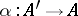the mappingis surjective. Every injective subobjectof an objectis a retract of. A product of injective objects is an injective object. If every object inis isomorphic to a subobject of an injective object in, one says thatis a category with enough injective objects (e.g., a Grothendieck category has this property). In such categories an object is injective if and only if it is a direct summand of any object containing it. For the objects of such categories one can construct resolutions consisting of injective objects (injective resolutions). This makes it possible to develop homological algebra in these categories.

In locally Noetherian categories (cf. Topologized category) a direct sum of injective objects is an injective object, and each injective object is isomorphic to a direct sum of indecomposable injective objects; this representation is moreover unique . Ifis the category of modules over a Noetherian commutative ring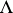, then the indecomposable injective modules are the injective hulls of the fields of fractions of the quotient rings, whereis an arbitrary prime ideal in.

## Contents

### Examples.

1) The category of Abelian groups has enough injective objects. These objects are the complete (divisible) groups.

2) The category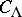of right-modules contains enough injective objects (cf. Injective module).

3) The category of sheaves of modules on a ringed topological space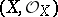contains enough injective objects. Examples of such objects are sheavesall stalks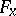of which are injective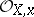-modules. Ifis a scheme, the converse statement holds for quasi-coherent-modules: Every stalk of an injective sheaf is an injective-module.

How to Cite This Entry:
Injective object. Encyclopedia of Mathematics. URL: http://encyclopediaofmath.org/index.php?title=Injective_object&oldid=15500
This article was adapted from an original article by I.V. Dolgachev (originator), which appeared in Encyclopedia of Mathematics - ISBN 1402006098. See original article